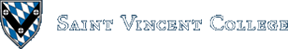Computing & Information Systems    DepartmentSearch CIS Site      Tutorials

## Software Design Using C++

### What is a Table?

A hash table is one way to create a table (sometimes called a dictionary). Typical operations are Empty, Insert, and Retrieve. The idea is that a table is a place to store data records, each containing a key value as well as other data fields (or field), so as to easily retrieve the data later by supplying a key value. The Retrieve function supplies the entire matching record of data.

### What is a Hash Table?

One way to create a table, either on disk or in main memory, is to use a hash table. The goal of a hash table is to get Theta(1) lookup time, that is, constant lookup time. Rather than having to proceed through a Theta(n) sequence of comparisons as in linear search, or a Theta(lg n) sequence of comparisons as in binary search, a hash table will usually complete a lookup after just one comparison! Sometimes, however, two comparisons (or even more) are needed. A hash table thus delivers (almost) the ideal lookup time. The trade-off is that to get this great lookup time memory space is wasted. This may well be a good trade-off, however, as memory is cheap.

The idea is that we store records of data in the hash table, which is either an array (when main memory is used), or a file (if disk space is used). Each record has a key field and an associated data field. The record is stored in a location that is based on its key. The function that produces this location for each given key is called a hash function.

Let's suppose that each key field contains an integer and the data field a string (array of characters type of string). One possible hash function is hash(key) = key % prime.

Recall that % is the mod operator that gives the remainder after integer division. The constant prime holds some prime number that is the length of the table. (For technical reasons, a prime number works better.) Perhaps we are storing our hash table in an array and use prime = 101. This means that our table can hold up to 101 records, at indices 0 through 100. If we want to insert 225 into the table we compute hash(225) as 225 % 101 = 23 and store the record containing key value 225 at index 23 in the array. Of course, if this location already contains a record, then we have a problem (called a collision). More on collisions later!

If we go to look up the record with key 225, we again calculate hash(225) and get 23. We go to index 23, check that the record at that position has key value 225, and copy that record. There it is: one comparison and we completed our lookup. The situation is even more dramatic when using a file, since disk access is so much slower and every new record that has to be read adds appreciably to the lookup time. Here the hash function values are record numbers not array indices, but it works very much the same. To look up 225, we find hash(225) = 23, read record 23 from the file, check that this record does indeed have key value 225 and we are done! Only one record had to be read!

### Collisions

When inserting items into a hash table, it sometimes happens that an item to be inserted hashes to the same location as an item already in the table. (Note that some method is needed to distinguish locations in use from unused locations.) The new item typically is inserted somewhere else, though we must be sure that there is still a way (and a fairly fast way) to look up the item. Here are several ways of handling collisions:

#### Rehash function

A second function can be used to select a second table location for the new item. If that location is also in use, the rehash function can be applied again to generate a third location, etc. The rehash function has to be such that when we use it in the lookup process we again generate the same sequence of locations. Since the idea in a hash table is to do a lookup by only looking at one location in the table, rehashing should be minimized as much as possible. In the worst case, when the table is completely filled, one could rehash over and over until every location in the table has been tried. The running time would be horrible!

#### Linked list approach (separate chaining)

All items that hash to the same location can be inserted in a linked list whose front pointer is stored in the given location. If the data is on disk, the linked list can be imitated by using record numbers instead of actual pointers. On disk, the hash table file would be a file of fake pointers (record numbers). Each record number would be for a record in another file, a file of nodes, where each node has a next field containing the number of the next record in the list.

#### Linked list inside the table (coalesced hashing)

Here each table entry is a record containing one item that hashed to this location and a record number for another item in the table that hashed to this location, which may have the record number of another that hashed to the same spot, etc. We are again using a linked list, but this list is stored within the table, whereas in the method above the list was external to the table, which only stored the front pointer for each list. Items beyond the first may be stored in an overflow area of the table (sometimes called the cellar) so as not to take up regular table space. This is the approach that I took in the example program that goes with this section. It is recommended that the overflow area take only about 15% of the space available for the table (the regular table area and overflow area combined).

#### Buckets

Sometimes each location in the table is a bucket which will hold a fixed number of items, all of which hashed to this same location. [Some authors use size 1. That is, each bucket only holds 1 item. However, we assume here that the size is bigger than 1.] This speeds up lookups because there is probably no need to go look at another location. However, a bucket could fill up so that new insertions that hash to this location will need some other collision handler, such as one of the above methods.

### Example Using Linear Probing

A simple rehash function is rehash(k) = (k + 1) % prime, where prime is the prime number giving the maximum number of items in the table. This is sometimes called linear probing, since when an item hashes to a location k that is already in use, the rehash function then tells us to try k + 1, the next location (unless k + 1 would be off the end of the table, in which case the rehash function sends us back to the start of the table at index 0). The next location tried would normally be k + 2, then k + 3, etc. Trying successive locations like this is simple, but it leads to clustering problems. When several items hash to the same location they build up a cluster of items that are side-by-side in the table. Any new item that hashes to any of these locations has to be rehashed and moved to the end of the cluster. Insertions to these locations tend to be slower as do lookups of the data stored there. This is because we are getting away from our goal of checking one location and finding our data item. The number of rehashes needed should be kept as small as possible. In the worst case, a table that is full except for one location just before the cluster would require Theta(n) rehashes as successive locations are tried (with wrap-around to index 0) until that one empty location is found.

Suppose we use integer key values, prime = 5, and the following functions:

 `````` hash(key) = key % prime; rehash(k) = (k + 1) % prime; ``````

If we insert a record with key value of 27, we compute hash(27) = 2 and thus get the following table. Nothing is shown in the data field in order not to complicate the drawing. The -1 in various key fields is used to show that this location is empty.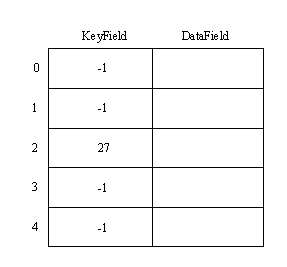Next, suppose we insert a record with key value 99. We compute hash(99) = 4, and so insert at index 4, giving: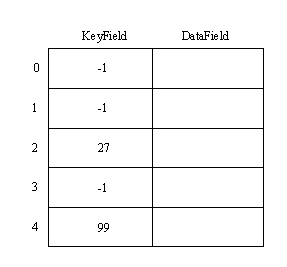Now, let's insert a record with key value 32. Since hash(32) = 2, we try location 2. It is in use, since the key value already stored there is not -1. We then compute rehash(2) = 3 and check location 3. It is empty, so we insert at index 3 as shown below.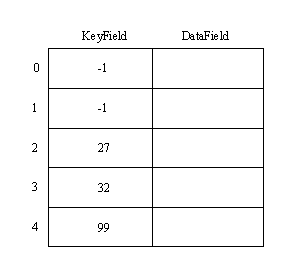Suppose we now try to look up the 32. Since hash(32) is 2, we check location 2. The key there does not match 32, so we know we must try the rehash function. Since rehash(2) = 3, we try location 3. The key value at index 3 matches, so we copy record 3. (Note that the lookup operation follows the same "rehash path" that was used when the record was inserted in the first place.)

Now suppose we try to look up 18. Since hash(18) = 3, we try index 3, but the key value there does not match. So, we try rehash(3) = 4, but the key value at index 4 does not match either. Next we try rehash(4) = 0, but this location has key -1, which indicates an empty location. We stop searching as soon as we reach an empty location and conclude that 18 is NOT FOUND in the table.

Next, let's insert 77. Since hash(77) = 2 we try index 2, but find it occupied. Thus we try rehash(2) = 3, but that location is in use. Next we try rehash(3) = 4, but that location is in use as well. Finally, rehash(4) = 0 gives us an empty location. We insert 77 at index 0.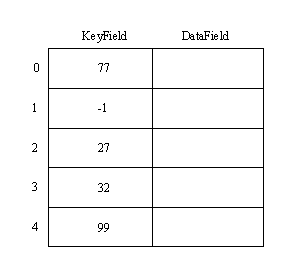Note the long rehash path used in inserting the 77. It will be the same long path used when looking up 77 later. The cluster starting at index 2 and extending to index 4 (and now back to index 0) is getting in the way of our goal of fast lookup. This is one reason that hash tables are deliberately left somewhat empty. That helps to eliminate the need for rehashing for most lookups and to reduce the number of rehash attempts needed in the other lookups. In the above example, note that inserting one more item would fill the table. Having a hash table that full is not desirable. Some check should be made to make sure, however, that any attempt to insert into a full table is rejected immediately. There is no need to rehash to every location in the table before concluding that there is no room; just have a counter and check that.

### Deleting

How one does deletion depends on the design of the hash table. If some sort of linked list was used, then it amounts to a deletion of a node from a linked list. If a design like that in the example above was used, then one just marks a deleted location as "deleted". In our example we could use a flag of -2 to stand for "deleted". For example, let's delete the record containing 32. First we do a lookup to find the location in the usual way: hash(32) = 2, rehash(2) = 3. We mark the table as follows: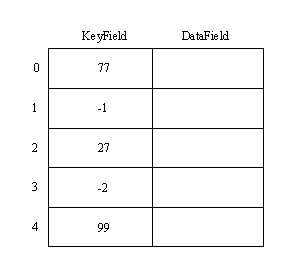Does this mess up our lookups? Looking up 27 or 99 works normally, since each of these hashes directly to the correct location. Let's try 77. Since hash(77) = 2, we try index 2, but find no match. Then rehash(2) = 3, but location 3 is marked as deleted. Although we stop a lookup when we reach an empty location (-1), it is clear that we must continue when reaching a deleted location (-2). Thus we try rehash(3) = 4, but again have no match. Finally, rehash(4) = 0 gives us the location of the desired 77. Lookups, then, have to continue until we find a match, an empty location, or until we have tried every location in the table (for a full table -- if we were crazy enough to produce such a thing).

### Hash Functions

A good hash function should be quick to compute, produce all possible indices from 0 to prime - 1, and should minimize collisions. For integer keys, hash(key) = key % prime is one simple hash function. Hashing by nonlinear table lookup is a more advanced method and one that is fast if properly implemented. For string keys, some method of converting the string to an integer is usually used, followed by taking result % prime to cut the integer value down to size. One also needs to worry about integer overflow. The hash value should ideally depend on every bit of the key. It is not good to ignore part of the key as that may lead to several keys that hash to the same location.

Here is one method for strings. Let's suppose that the strings only contain the letters of the alphabet, in upper case. In other words, we have the letters A through Z. We can translate the individual letters to the integers 0 through 25. Then we treat the string as a base 26 number. For example, CPU would be the base 26 number with digits 2, 15, 20. The decimal value of this number is 2(26^2) + 15(26) + 20. All computations are done % prime, both to avoid overflow, and because the final hash value must fit the range 0 to prime - 1. For example, if prime = 101, we would get 26^2 % 101 = 676 % 101 = 70. Then 2(26^2) % 101 = 39. The 15(26) % 101 = 390 % 101 = 87. Thus we get (39 + 87) % 101 = 126 % 101 = 25. The final hash value is thus (25 + 20) % 101 = 45. If your strings include more of the ASCII character set than this, you might want to use the ASCII value of each letter instead of mapping A to 0, B to 1, etc.

A folding method is sometimes used to hash integers. Here the key is chopped into two or more parts and the parts recombined in some easy manner. For example, suppose the keys are 9 digit longs, such as 542981703. Chop this into 3 equal-length pieces and add them together: 542 + 981 + 703, taking the sum % prime to cut the answer down to size.

Above it was stated that the size of the hash table should be prime. A particularly bad choice for the size of the table is a small power j of 2 or a small power i of 10. In the first case, say with j = 8, any binary numbers that have the same low order 8 bits would hash to the same location. Similarly with the second case, if i = 3 say, then any decimal numbers with the same last 3 digits would hash to the same location.

If you use something like the above hash function for strings and aren't careful about the size of the hash table, there can be significant problems. Suppose we convert each letter to its ASCII value and treat each string as a base 256 number. Further suppose that we choose 255 as the size of the hash table. Then hash("mac") = [109(256^2) + 97(256) + 99] % 255 since the ASCII values of m, a, and c are 109, 97, and 99 respectively. However, since 256 mod 255 = 1, that hash value simplifies to (109 + 97 + 99) % 255. If we permute the letters of our string, perhaps to "cam", we get hash("cam") = [99(256^2) + 97(256) + 109] % 255 = (99 + 97 + 109) % 255. Thus hash("mac") = hash("cam"). Any permutation of these 3 letters generates the same hash value, and inserting any two of them into your hash table produces a collision.

Note that if the radix happened to be 42 instead of 256 and we used a hash table size of 1 less, giving 41 which is prime, we would still have the above problem that permutations of the same letters hash to the same location. So, we actually need the table size p to be more than simply a prime. Some authors (such as Gregory L. Heileman in Data Structures, Algorithms, and Object-Oriented Programming, McGraw Hill (1996), p 222) say that you should choose a prime p such that p does not divide any small power of the radix plus or minus any small non-negative integer. Radix 42 and p = 41 fail to satisfy that condition since 41 = 42^1 - 1, and 41 certainly divides 41.

In general, finding a good hash function is both important and tricky. It is important because it strongly affects the efficiency of your hash table. It is tricky because there are so many conditions to satisfy and complications to avoid.

### A Simple Hash Table Example (New in 2021)

See SimpleHashTable.cpp for an easy implementation of a hash table. This one stores short strings and has a new linked list for each set of strings that hash to the same location.

This program is deliberately kept simple so that you have an easy example to start with. It does not use an object-oriented approach (though a few of the items in the program, such as the strings) are objects. It does not use the STL (standard template library). It also has all of the code in one file. This is to make this example easy to follow. The linked lists add some complexity, but linked lists are usually learned long before hash tables are encountered.

Another feature of this example is that it is an example that is designed to show you what it is doing. After prompting you for the strings (such as the names of the grocery items you want to purchase) to put into the hash table, the program allows you to enter strings to be looked up in the table. For each such string, it tells you the array index where the linked list is that contains your string (or it tells you that it is not in the table if that is the case). Finally, the program gives you the option of doing a dump to the screen of all of the data in your hash table. That way you can see all of the items that hashed to any of the indices. Thus the program was designed to be more educational than practical.

### A Hash Table Example

 NOTE: This example has not been revised in many years. Some changes would probably be needed to get it to run today. itemtype.h table.h (sets up an abstract base class for tables) hash.h (derives a hash table class) hash.cpp hash.txt (text file of data to use) hashmake.cpp (creates a hash table) hashread.cpp (reads data from a hash table)
There are two programs in this example. Both of them use the `.h` files and the `hash.cpp` file. The main function for the first program is in `hashmake.cpp`. This program builds a hash table from the data in the `hash.txt` file. The hash table is placed in a file named `hash.dat`. The second program has its main function in the `hashread.cpp` file. This program allows the user to interactively look up data in the hash table. It reads this data from the `hash.dat` file, of course. Note that this example uses coalesced chaining (a linked list inside the table with all items after the first in an overflow area of the table). Let's see how it works.

The file is organized as a binary file of records. Each record has an Info and a Next field. The Next field will contain the record number for the next record in the linked list (when we have to chain nodes together) or a -1 as a fake NULL pointer. Record 0 (the first record in the file) is not used to hold node data at all. Instead, it holds in Info.KeyField the number of items in the table. That way this count is stored permanently, along with the file. When an empty table is first created, the entire file is filled with empty nodes. Thus, the special record 0, followed by empty nodes 1 through MaxFile = 150 are written to the file. These nodes are marked as empty by placing 0 in the KeyField locations. (This assumes that 0 is not a normal KeyField value.) The regular part of the table includes nodes 1 through Prime = 101, and the overflow area is from node 102 to node MaxFile = 150. A field of the HashTableClass object, called OverflowIndex, is used to keep track of the next free record for overflow use. (Thus OverflowIndex is initially set to 102. If it reaches 151, the overflow area is full.)

The following is a drawing of a HashtableClass object, showing the five data fields. Note that the OpenMode field contains the character r or w, indicating whether the table has been opened in read or write mode. NodeSize is the number of bytes per node. Since this value is often needed, it is convenient to store it in a field of the object. The DataFile field is an fstream object containing information about the file where the table's data is held. The details of an fstream object are beyond what we will study here, but include information such as the current position in the file.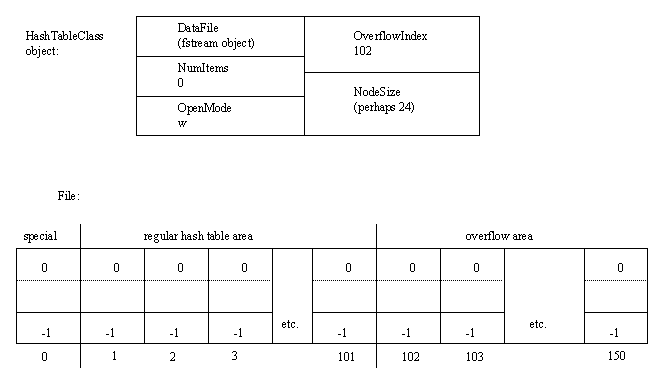Note that when we open a hash table in write mode, we really open the underlying data file, `hash.dat` for both input and output. We also specify that it is a binary file. Depending on your compiler, you may need to use `ios::trunc` as was done here. This tells the program to truncate (erase) any existing file by the same name when the open is carried out.

The data for this program is read from a text file, hash.txt, that contains an integer (long) followed by a name (character string), with these items on separate lines. To make it easy to read in the strings, no spaces are used. Rather, underscores are used where spaces would normally appear, as in Bill_Clinton, the name that goes with key value 7.

If you run the `hashmake` program from within the Visual C++ environment you need to be sure that the hash.txt file is in the project directory for this program. If instead you want to run the program by double clicking on the `hashmake.exe` file in MyPrograms, then you want hash.txt to be in the same directory (usually the Debug directory) that contains `hashmake.exe`.

The hash function used is hash(key) = (key % prime) + 1. The + 1 is there because record 0 is not used for table data. Thus the hash function has to produce the integers from 1 to prime. When inserting an item in the table, the item's key value is hashed to produce the desired location. The record at that location is read and its Info.KeyField examined to see if it is 0 (which indicates an unused record). If so, a record containing the item is written to the file at this location. If, however, the location produced by the hash function is in use, we insert the item at the location given by OverflowIndex (unless OverflowIndex > MaxFile, which means that the overflow area is full). The new record written to this location contains item and a Next field containing the record number from the Next field of the record at the location produced by the hash function. Finally, the record at the location produced by the hash function is rewritten so as to contain in its Next field OverflowIndex, the location of our newly inserted node. OverflowIndex is then incremented, of course. Note that after writing the above 2 records to the file, we have correctly linked the new record into the linked list of records hashing to that same location, with the new record added as the second record of the list.

The following drawing shows our hash table after 4 items have been inserted. One item hashed to location 1 and was inserted there. The other 3 items hashed to location 3, so that two of them had to be placed in the overflow area. Note how these three are linked together in a linked list via the Next field values. Also note how the number of items (4 in this case) is stored in the NumItems field in the object, as well as in node 0 in the file. (However, node 0 is only updated when we finish using the table. This is so that we do not waste time repeatedly writing to node 0 just to update that counter.)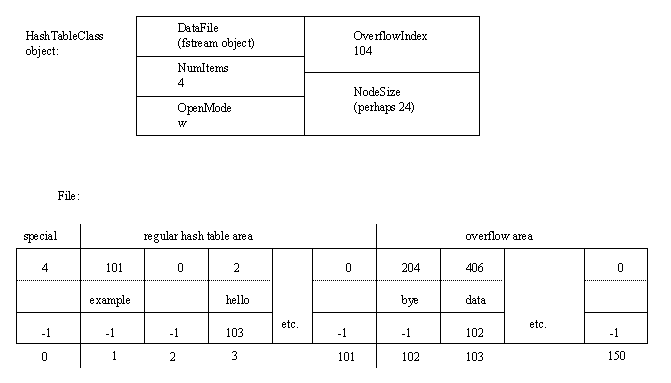Next, let's consider the second program in this example. It allows us to interactively look up data from the hash table. When a table is first opened for reading, the special record 0 is read in so that the number of items in the table can be discovered by examining Info.KeyField. To do a lookup of a key value, we first compute hash(key) and read in the record with that number as its location. If the key in this record matches, we are done. If not and the Next field of this record contains -1 (used as a fake NULL pointer), then this key is not in the table. Otherwise, the Next field number is the location for the next record that hashes to this location. We read this new record (which will be in the overflow area) and see if the key matches the target key. If not, we check the Next field. If we have -1 we report that the key cannot be found. Otherwise, we read the record whose location is given by this Next field, check its key value, etc. The search ends when we either find a matching key or get a -1 as the Next pointer.

### More on Rehash Functions

A good rehash function should produce all of the allowed indices (usually 0 to prime - 1). A rehash function that skipped some numbers might cause you to not be able to insert an item because the empty locations are at indices that the rehash function cannot produce. A good rehash function should also try to avoid clustering as much as possible, whether clustering that occurs when items hash to the same location, or when items that hash to different locations compete for the same locations in their rehash paths.

One example rehash function is rehash(k) = (k + m) % prime for some constant m which is less than prime. Linear probing is just one case of this (when m = 1). This type of rehash function tends to have a lot of problems with clustering.

Another is rehash(k, try) = (k + try) % prime, where try indicates whether this is the first (try = 1), second (try = 2), etc. attempt to rehash. Suppose, for example, that we have hashed to location 4 and found it in use. We then use rehash(4, 1) to get 5 % 5 = 0 (where I have assumed that we are using prime = 5). If location 0 is also in use, we use rehash(0, 2) = 2, etc. Note that we skipped location 1. This helps to avoid some clustering, but does not eliminate all of it.

One recommended scheme that gets rid of clustering as much as possible, is double hashing. This method uses two functions h1 and h2. Suggested functions are h1(key) = key % prime and h2(key) = 1 + (key % (prime - 2)). One then uses hash(key) = h1(key). If rehashing is needed, first compute h2(key) and store it in a variable so that you do not need to recompute it. Then use rehash(k, key) = (k + h2(key)) % prime, where k is the table location just tried. Since the rehash function depends on the key value, different values which hash to the same location in the table will probably not follow the same rehash path, thus eliminating that kind of clustering.

Note that this type of rehash function, or the simpler rehash(k) = (k + m) % prime, provide another reason to use a prime for the table size. Here is a simple example. Suppose prime = 7, m (or h2(key)) is 4, and hash(key) = 2. Then the rehash path takes us to these indices: (2 + 4) % 7 = 6, (6 + 4) % 7 = 3, (3 + 4) % 7 = 0, (0 + 4) % 7 = 4, (4 + 4) % 7 = 1, (1 + 4) % 7 = 5, (5 + 4) % 7 = 2. Note that we hit every index in the table before returning to the starting index of 2. However, if we would use a table size of 6, which is not prime, the rehash path becomes: (2 + 4) % 6 = 0, (0 + 4) % 6 = 4, (4 + 4) % 6 = 2. We are back at the starting index and only hit every other spot in the table with our rehash path. Having fewer locations in the rehash path gives us fewer chances at finding an open slot to insert a new item that happened to hash to the already used spot 2.

### Efficiency

According to Tenenbaum and Augenstein's text, the following table gives the approximate average number of probes of a hash table for both successful and unsuccessful lookups when using linear probing as well as double hashing. Note that the load factor is defined as n / prime where n is the number of items in the table and prime is the size of the table. Thus a load factor of 1 means that the table is full. In constructing the table it was assumed that prime was a large number, not a small one like the 101 in the above example program.

load factor successful lookup unsuccessful lookup
linear double linear double
0.50 1.50 1.39 2.50 2.00
0.75 2.50 1.85 8.50 4.00
0.90 5.50 2.56 50.50 10.00
0.95 10.50 3.15 200.50 20.00

Recall that the goal of a hash table is to do each lookup with just 1 probe, or very close to that. The double hashing scheme with a load factor of 50% is pretty close to achieving that. The 2 probes for an unsuccessful lookup is a little long, but not too bad. Note that the linear probing scheme tends not to perform well, especially as the table fills up. When the table is 95% full, the linear scheme gives really bad unsuccessful lookup times, but the double hashing scheme might still be acceptable.

There are formulas and tables available for hash tables using some of the other collision-resolution schemes. For example, in separate chaining, where items that hash to the same location are stored in a linked list, lookup time is measured by the number of list nodes that have to be examined. For a successful search, this number is 1 + lf / 2, where lf is the load factor. Because each table location holds a linked list, which can contain a number of items, the load factor can be greater than 1, whereas 1 is the maximum possible in an ordinary hash table. For a load factor of 1, the average number of probes is 1.5, which is pretty close to our ideal.

### References:

 Data Structures & Program Design, 2nd ed. Robert L. Kruse. Prentice-Hall (1987). There is also a third edition (1994) as well as a new text, Data Structures and Program Design in C++, authored by Kruse and Alexander J. Ryba (1999). Data Structures Using Pascal, 2nd ed. Aaron M. Tenenbaum, Moshe J. Augenstein. Prentice-Hall (1986). Data Structures with C++. William Ford, William Topp. Prentice-Hall (1996).
Back to the main page for Software Design Using C++

Author: Br. David Carlson with contributions by Br. Isidore Minerd
Last updated: July 21, 2021
Disclaimer